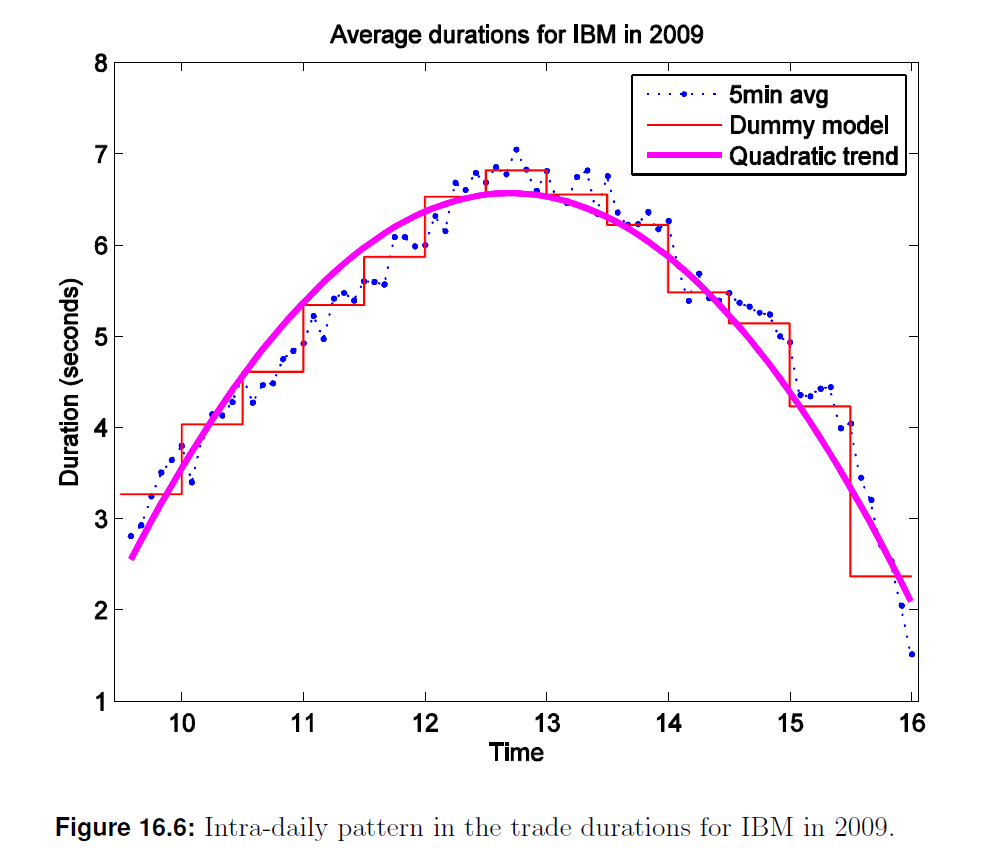21
Jun 20

## Solution to Question 3 from UoL exam 2019, zone A

(a) Define the concept of trade duration in financial markets and explain briefly why this concept is economically useful. What features do trade durations typically exhibit and how can we model these features? [25 marks]

High frequency traders (HFT) may trade every millisecond. Orders from traders arrive at random moments and therefore the trade times are not evenly spaced. It makes sense to model the differences${x_j} = TIME_j - TIME_{j - 1}$

between transaction times. (The Guide talks about differences between times of returns but I don’t like this because on small time frames people are interested in prices, not returns.) Those differences are called durations. They are economically interesting because 1) they tell us something about liquidity: periods of intense trading are generally periods of greater market liquidity than periods of sparse trading (there is also after-hours trading between 16:00 and 20:30, New York time, when trading may be intense but liquidity is low) and 2) durations relate directly to news arrivals and the adjustment of prices to news, and so have some use in discussions of market efficiency.

The trading session in the USA is from 9:30 to 16:00, New York time. Durations exhibit diurnality (that is, intraday seasonality): transactions are more frequent (durations are shorter) in the first and last hour of the trading session and less frequent around lunch, see Figure 16.6 from the Guide.Higher frequency in the first hour results from traders rebalancing their portfolios after overnight news and in the last hour – from anticipation of news during the next night.

The main decomposition of durations is${x_j} = {s_j}x_j^*,$
so$\log {x_j} = \log {s_j} + \log x_j^*,$$\log {s_j} = \sum\limits_{i = 1}^{13} {\beta _j}{D_{i,j}}$$\log {s_j} = \gamma _0 + \gamma _1HR{S_j} + \gamma _2HRS_j^2.$

In the first equation${s_j}$ is the diurnal component and$x_j^*$ is called a de-seasonalized duration (it has not been defined here). The second follows from the first.

I am not sure that you need the third equation. The fourth equation is used below. In the third equation$\log {s_j}$ is regressed on dummies of half-hour periods (there are 13 of them in the trading session; the constant is not included to avoid the dummy trap). In the fourth equation it is regressed on the first and second power of the time variable$HRS_j$, which measures time in hours starting from the previous midnight. This is called a polynomial regression. Both regressions can capture diurnality.

(b) Describe the Engle and Russell (1998) autoregressive conditional duration (ACD) model. [25 marks]

Instead of the duration model considered in part (a) Engle and Russell suggest the ACD model${x_j} = {s_j}x_j^*,$$x_j^* = {\psi _j}{\varepsilon _j},$
where$\log {s_j} = {\beta _0} + {\gamma _1}HR{S_j} + {\beta _2}HRS_j^2,$$x_j^\star = \frac{{x_j}}{{s_j}},\ {\varepsilon _j}|{F_t}\sim i.i.d.(1),$$\psi _j = \omega + \beta \psi _{j - 1} + \alpha x_{j - 1}^*,\,\,\omega > 0,\,\,\alpha ,\beta \ge 0$   (1)

The first decomposition is the same as above. The second equation decomposes the de-seasonalized duration into a product of deterministic and stochastic components. To understand the idea, we can compare (1) with the GARCH(1,1) model:$y_{t + 1} = {\mu _{t + 1}} + {\varepsilon _{t + 1}},$${\varepsilon _{t + 1}} = {\sigma _{t + 1}}{v_{t + 1}},$${v_{t + 1}}|{F_t}\sim F(0,1),$$\sigma _{t + 1}^2 = \omega + \beta \sigma _t^2 + \alpha \varepsilon _t^2.$   (2)

Equations (1) and (2) are similar. The assumptions about the random components are different: in (1) we have$E{\varepsilon _j} = 1$, in (2)$E{v_j} = 0$. This is because in (2) the epsilons are deviations from the mean and may change sign; in (1) the epsilons come from durations and should be positive. To obtain the last equation in (1) from the GARCH(1,1) in (2) one has to make replacements$\sigma _t^2\sim {\psi _j},\ \varepsilon _t^2 = {({y_{t + 1}} - {\mu _{t + 1}})^2}\sim x_{j - 1}^*$. (3)

This is important to know, to understand the comparison of the ML method for the two models below.

(c) Compare the conditions for covariance stationarity, identification and positivity of the duration series for the ACD(1,1) to those for the GARCH(1,1). [25 marks]

Those conditions for GARCH are

Condition 1:$\omega > 0,\ \alpha,\beta \ge 0,$ for positive variance,

Condition 2:$\beta = 0$ if$\alpha = 0,$ for identification,

Condition 3:$\alpha + \beta < 1,$ for covariance stationarity.

For ACD they are the same, because both are essentially ARMA models.

(d) Illustrate the relationship between the log-likelihood of the ACD(1,1) model and the estimation of a GARCH(1,1) model using the normal likelihood function. [25 marks]

Because of the assumption$E{\varepsilon _j} = 1$ in (1) we cannot use the normal distribution for (1). Instead the exponential random variable is used. It takes only positive values; its density is zero on the left half-axis and is an exponential function on the right half-axis:$Z\sim Exponential(\gamma ),$

so$f(z|\gamma ) = \frac{1}{\gamma }\exp\left(-\frac{z}{\gamma } \right)$ and$EZ = \gamma .$

Here$\gamma$ is a positive number and$f$ is the density. We take$\gamma= 1$ as required by the ACD model. This implies$Ex_j^* = {\psi _j}E{\varepsilon _j} = {\psi _j}$ so$x_j^*$ is distributed as$Exponential({\psi _j}).$ Its density is$f(x_j^*|\psi _j)=f(x_j^*|\psi _j(\omega,\beta,\alpha))=\frac{1}{\psi _j}f\left(-\frac{x_j^*}{\psi _j}\right).$

The rest is logical: plug$\psi_j$ from the ACD model (1), then take log and then add those logs to obtain the log-likelihood. A. Patton gives the log-likelihood for GARCH, whose derivation I could not find in the book. But from (3) we know that there should be similarity after replacement$\sigma _t^2\sim{\psi _j},\ x_{j - 1}^*\sim{({r_t} - {\mu _t})^2}$. To this Patton adds that the GARCH likelihood is simply a linear transformation of the ACD likelihood.